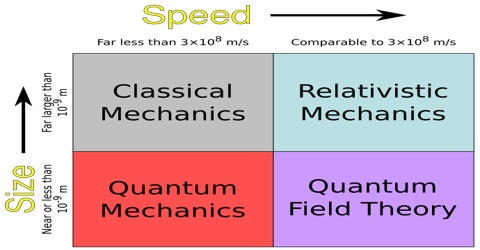# Newtonian Mechanics: Foundation of Modern Physics

Newtonian mechanics: Foundation of Modern Physics

Mechanics which has been created and developed on the basis of Newton’s laws motion is called Newtonian Mechanics or Classical Mechanics. By the help of this mechanics motion of bodies on earth and motion of stars and planets in the vast endless sky can be analyzed. By applying Newton’s laws of motion we can get accurate solutions of the motion of these bodies. Newtonian Mechanics or Classical mechanics has achieved remarkable success in analyzing the motion of these bodies. For this reason, Newtonian mechanics is said to have laid the foundation of modem physics.

It has been assumed in Newtonian mechanics that mass and length of a body do not depend on the velocity of the body. Furthermore, it has been assumed that action or performance of measuring instruments is not influenced by their motion. In this mechanics both space and time have been considered to be constant and are not relative with respect to anything else. In Newton’s first law of motion only inertia can be correctly expressed with respect to a reference. For this reason, in Newtonian mechanics a particular absolute reference of rest is to be considered.

But scientist Einstein after extensive research came to the conclusion that no absolute rest is there in the universe. All are in motion, but it may appear that a particular body is at rest with reference to another body. He further proved that laws of physics expressed in any frame at reference remain unchanged. This is the fundamental concept of the theory of relativity of Einstein. In order to make it applicable in all cases, scientist Einstein changed many Newtonian equations of motion. He proved these changes by different experiment. One of these is the fact that the mass of a body depends on speed. So, the acceleration that occurs due to the application of force does not remain constant, i.e., acceleration of a body depends on its speed. Besides, length of a body and time interval also depends on motion. Experimental results of different measurement also depend on motion. Einstein’s theory of relativity has generated many new concepts in physics and has changed many old ideas. Newtonian mechanics is not effective in those cases where velocity of bodies is almost close to the velocity of light. In analyzing this type of high speed or motion relativistic mechanics is needed.

In analyzing motions of atoms and molecules Newtonian mechanics is not effective. Quantum mechanics is needed to analyze the motion of such tiny particles. But that does not mean that classical mechanics has become obsolete.